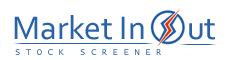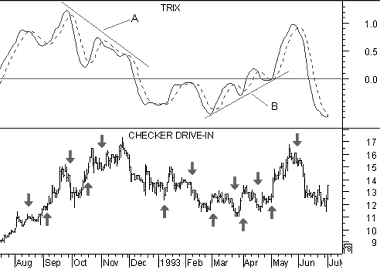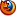Please enable JavaScript to view this page content properly Log In | Sign Up
 TRIX
T R I X

Overview

TRIX is a momentum indicator that displays the percent rate-of-change of a triple exponentially smoothed moving average of the security's closing price. It is designed to keep you in trends equal to or shorter than the number of periods you specify.

Interpretation

The TRIX indicator oscillates around a zero line. Its triple exponential smoothing is designed to filter out "insignificant" cycles (i.e., those that are shorter than the number of periods you specify).

Trades should be placed when the indicator changes direction (i.e., buy when it turns up and sell when it turns down). You may want to plot a 9-period moving average of the TRIX to create a "signal" line (similar to the MACD indicator, and then buy when the TRIX rises above its signal, and sell when it falls below its signal.

Divergences between the security and the TRIX can also help identify turning points.

Example

The following chart shows Checker Drive-In, its 12-day TRIX (solid line), and a 9-day "signal" moving average of the TRIX (dotted line).I drew "buy" arrows when the TRIX rose above its signal line and drew "sell" arrows when it fell below its signal line. This method worked well when prices were trending, but it generated numerous false signals when prices were moving sideways.

A bearish divergence occurred when the TRIX was falling (trendline "A") while prices rose. Prices subsequently corrected. Similarly, a bullish divergence occurred when the TRIX was rising (trendline "B") while prices were falling. Prices subsequently rallied.

Calculation

To calculate the TRIX indicator:

1. alculate an n-period exponential moving average of the closing prices.

2. Calculate an n-period exponential moving average of the moving average calculated in Step #1.

3. Calculate an n-period exponential moving average of the moving average calculated in Step #2.

4. Calculate the 1-period (e.g., 1-day) percent change of the moving average calculated in Step #3.

 Best viewed in